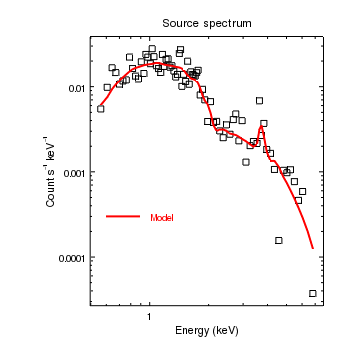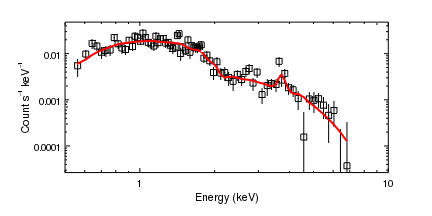# Gallery: Overplotting curves

## 1) Two curves in the same plot

A plot can contain multiple objects; in this example we show how to add a second curve to a plot and to annotate it with both a line and a label.Version: Postscript; PDF
```crv = ChipsCurve()
crv.line.style = "noline"
crv.symbol.style = "square"
crv.symbol.size = 3
crv.symbol.fill = False
crv = ChipsCurve()
crv.symbol.style = "none"
crv.line.color = "red"
crv.line.thickness = 2
log_scale()

set_plot_xlabel("Energy (keV)")
set_plot_ylabel("Count s^{-1} keV^{-1}")
set_plot_title("Source spectrum")

set_xaxis(["offset.perpendicular",35])
set_plot(["leftmargin",0.25])
set_yaxis(["offset.perpendicular",45])

```

Two curves are created - in this case from different blocks in the same data file; the first, from the data block, is displayed as open squares and the second curve, which is from the fit block, is drawn as a solid red line.

Labels are added to the plot axes and title (these labels can include a subset of LaTeX commands), and the plot re-arranged to make all the labels fit into the display area.

Finally, a line and label are added to the plot to label the second curve. In this example a single segment line is used, so only the start and end locations are given in the add_line call. The vertical alignment of the label is adjusted so that it is centered on the line

The info and info_current can be used to find out what objects have been created and which are current:

```chips> info()

Window [win1]
Frame [frm1]
Plot [plot1]   (0.25,0.215)  .. (0.90,0.90)
Border bottom [bx1]  top [bx2]  left [by1]  right [by2]
Curve [crv1]
X Axis [ax1]
Y Axis [ay1]
Curve [crv2]
Line [line1]
Label [lbl1]

chips> info_current()

Window [win1]
Frame [frm1]
Plot [plot1]
X Axis [ax1]
Y Axis [ay1]
Curve [crv2]
Line [line1]
Label [lbl1]
Coord Sys [Data]
Coord Sys ID [ds0.0.0.3]
```

## 2) Two curves in the same plot, one with error bars

Here we plot the same data as used in the Two curves in the same plot example, but this time we create a rectangular plot and also display error bars on the first curve.Version: Postscript; PDF
```add_window(6,3,"inches")
crv = ChipsCurve()
crv.line.style = "noline"
crv.symbol.style = "square"
crv.symbol.size = 3
crv.symbol.fill = False
crv = ChipsCurve()
crv.symbol.style = "none"
crv.line.color = "red"
crv.line.thickness = 2
log_scale()
limits(X_AXIS,0.5,10)

set_plot_xlabel("Energy (keV)")
set_plot_ylabel("Count s^{-1} keV^{-1}")

set_xaxis(["offset.perpendicular",35])
set_plot(["bottommargin",0.2])
set_yaxis(["offset.perpendicular",45])
```

As with other ChIPS objects, windows will be created automatically for you if needed (e.g. by a call to add_curve). In this example we explicitly create the window using add_window so that we can control the window size. We pick a rectangle 6 inches wide and 3 inches high; these sizes refer to the postscript/PDF output size and will only be approximate for the on screen display; the screen size depends on the dot-pitch of your monitor and can be set using the scalewidth and scaleheight window settings when the window is created.

Since a window exists, the first add_curve call will use it instead of creating a new one. Since the Data Model filter selects four columns from the file - namely x, y, dylo, and dyhi - the curve is plotted with asymmetric Y error bars.

Although in this case the window size was explicitly set, print_window can be made to create postscript and PDF plots of a different size by using some or all of the pagesize, pagewidth, pageheight, fittopage, and keepaspect export options. These can easily be changed from a GUI created by using the Right-Mouse-Button to select the 'Print' optionor by setting the printdialog attribute to True when calling print_window:

```print_window(["printdialog", True])
```